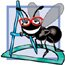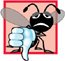# Recursion vs. Iteration

In the two previous sections, we studied two functions that easily can be implemented recursively or iteratively. This section compares the two approaches and discusses why the programmer might choose one approach over the other in a particular situation.

Both iteration and recursion are based on a control statement: Iteration uses a repetition structure; recursion uses a selection structure. Both iteration and recursion involve repetition: Iteration explicitly uses a repetition structure; recursion achieves repetition through repeated function calls. Iteration and recursion both involve a termination test: Iteration terminates when the loop-continuation condition fails; recursion terminates when a base case is recognized. Iteration with counter-controlled repetition and recursion both gradually approach termination: Iteration modifies a counter until the counter assumes a value that makes the loop-continuation condition fail; recursion produces simpler versions of the original problem until the base case is reached. Both iteration and recursion can occur infinitely: An infinite loop occurs with iteration if the loop-continuation test never becomes false; infinite recursion occurs if the recursion step does not reduce the problem during each recursive call in a manner that converges on the base case.

To illustrate the differences between iteration and recursion, let us examine an iterative solution to the factorial problem (Fig. 6.32). Note that a repetition statement is used (lines 2829 of Fig. 6.32) rather than the selection statement of the recursive solution (lines 2427 of Fig. 6.29). Note that both solutions use a termination test. In the recursive solution, line 24 tests for the base case. In the iterative solution, line 28 tests the loop-continuation conditionif the test fails, the loop terminates. Finally, note that instead of producing simpler versions of the original problem, the iterative solution uses a counter that is modified until the loop-continuation condition becomes false.

Figure 6.32. Iterative factorial solution.

(This item is displayed on pages 295 - 296 in the print version)

``` 1 // Fig. 6.32: fig06_32.cpp
2 // Testing the iterative factorial function.
3 #include
4 using std::cout;
5 using std::endl;
6
7 #include
8 using std::setw;
9
10 unsigned long factorial( unsigned long ); // function prototype
11
12 int main()
13 {
14 // calculate the factorials of 0 through 10
15 for ( int counter = 0; counter <= 10; counter++ )
16 cout << setw( 2 ) << counter << "! = " << factorial( counter )
17 << endl;
18
19 return 0;
20 } // end main
21
22 // iterative function factorial
23 unsigned long factorial( unsigned long number )
24 {
25 unsigned long result = 1;
26
27 // iterative declaration of function factorial
28 for ( unsigned long i = number; i >= 1; i-- )
29  result *= i;
30
31 return result;
32 } // end function factorial
```

 ``` 0! = 1 1! = 1 2! = 2 3! = 6 4! = 24 5! = 120 6! = 720 7! = 5040 8! = 40320 9! = 362880 10! = 3628800 ```

Recursion has many negatives. It repeatedly invokes the mechanism, and consequently the overhead, of function calls. This can be expensive in both processor time and memory space. Each recursive call causes another copy of the function (actually only the function's variables) to be created; this can consume considerable memory. Iteration normally occurs within a function, so the overhead of repeated function calls and extra memory assignment is omitted. So why choose recursion?

Software Engineering Observation 6.18Any problem that can be solved recursively can also be solved iteratively (nonrecursively). A recursive approach is normally chosen in preference to an iterative approach when the recursive approach more naturally mirrors the problem and results in a program that is easier to understand and debug. Another reason to choose a recursive solution is that an iterative solution is not apparent.

Performance Tip 6.9Avoid using recursion in performance situations. Recursive calls take time and consume additional memory.

Common Programming Error 6.26Accidentally having a nonrecursive function call itself, either directly or indirectly (through another function), is a logic error.

Most programming textbooks introduce recursion much later than we have done here. We feel that recursion is a sufficiently rich and complex topic that it is better to introduce it earlier and spread the examples over the remainder of the text. Figure 6.33 summarizes the recursion examples and exercises in the text.

Figure 6.33. Summary of recursion examples and exercises in the text.

Location in Text

Recursion Examples and Exercises

Chapter 6

Section 6.19, Fig. 6.29

Factorial function

Section 6.19, Fig. 6.30

Fibonacci function

Exercise 6.7

Sum of two integers

Exercise 6.40

Raising an integer to an integer power

Exercise 6.42

Towers of Hanoi

Exercise 6.44

Visualizing recursion

Exercise 6.45

Greatest common divisor

Exercise 6.50, Exercise 6.51

Mystery "What does this program do?" exercise

Chapter 7

Exercise 7.18

Mystery "What does this program do?" exercise

Exercise 7.21

Mystery "What does this program do?" exercise

Exercise 7.31

Selection sort

Exercise 7.32

Determine whether a string is a palindrome

Exercise 7.33

Linear search

Exercise 7.34

Binary search

Exercise 7.35

Eight Queens

Exercise 7.36

Print an array

Exercise 7.37

Print a string backward

Exercise 7.38

Minimum value in an array

Chapter 8

Exercise 8.24

Quicksort

Exercise 8.25

Maze traversal

Exercise 8.26

Generating Mazes Randomly

Exercise 8.27

Mazes of Any Size

Chapter 20

Section 20.3.3, Figs. 20.520.7

Mergesort

Exercise 20.8

Linear search

Exercise 20.9

Binary search

Exercise 20.10

Quicksort

Chapter 21

Section 21.7, Figs. 21.2021.22

Binary tree insert

Section 21.7, Figs. 21.2021.22

Preorder traversal of a binary tree

Section 21.7, Figs. 21.2021.22

Inorder traversal of a binary tree

Section 21.7, Figs. 21.2021.22

Postorder traversal of a binary tree

Exercise 21.20

Exercise 21.21

Exercise 21.22

Binary tree delete

Exercise 21.25

Printing treeC++ How to Program (5th Edition)
ISBN: 0131857576
EAN: 2147483647
Year: 2004
Pages: 627